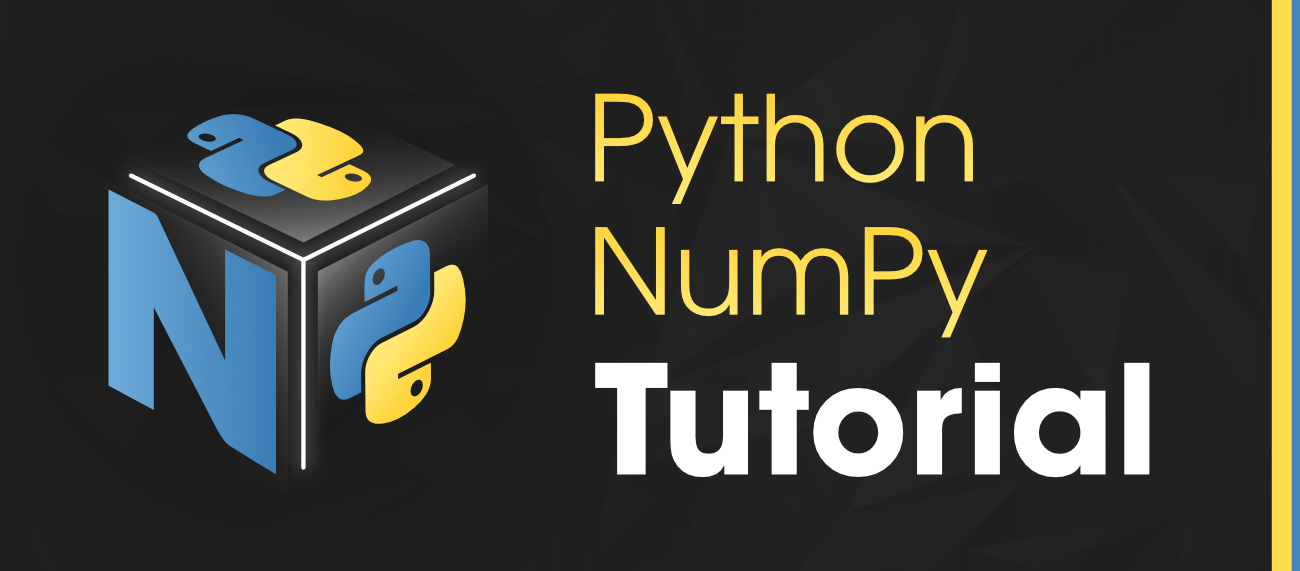# NumPy Tutorial – Python Library

NumPy is a general-purpose array-processing Python library which provides handy methods/functions for working n-dimensional arrays. NumPy is a short form for “Numerical Python“. It provides various computing tools such as comprehensive mathematical functions, and linear algebra routines.

• NumPy provides both the flexibility of Python and the speed of well-optimized compiled C code.
• Its easy-to-use syntax makes it highly accessible and productive for programmers from any background.

This NumPy tutorial helps you learn the fundamentals of NumPy from Basics to Advanced, like operations on NumPy array, creating and plotting random data sets, and working with NumPy functions.## Why Numpy ?

NumPy revolutionized the way we handle numerical data in Python. It is created to address the limitations of traditional Python lists when it comes to numerical computing. It is developed by Travis Olliphant in 2005.

NumPy provides a powerful array object that is both efficient and flexible. Its primary goal is to facilitate complex mathematical and scientific operations by introducing array-oriented computing capabilities. NumPy’s design allows for seamless integration with other scientific libraries, enabling faster execution of numerical tasks.

As a result, NumPy has become a cornerstone in the Python ecosystem, essential for data manipulation, machine learning, and scientific research.

### Installation of Numpy Using PIP

Open your command prompt or terminal and run the following command:

`pip install numpy`

## Python

 `import` `numpy as np ` ` `  `# Create two NumPy arrays ` `array1 ``=` `np.array([``1``, ``2``, ``3``]) ` `array2 ``=` `np.array([``4``, ``5``, ``6``]) ` ` `  `# Perform element-wise addition ` `result_addition ``=` `array1 ``+` `array2 ` ` `  `# Display the original arrays and the results ` `print``(``"Array 1:"``, array1) ` `print``(``"Array 2:"``, array2) ` `print``(``"Element-wise Addition:"``, result_addition)`

## Conclusion

From simple calculations to complex data manipulation, NumPy empowers you to tackle any numerical challenge, no matter the dimensionality. Its intuitive syntax and powerful functionality make you feel like you’re working with single numbers, even when manipulating vast datasets.

This Numpy tutorial has equipped you with the fundamental skills to:

• Grasp the fundamental concepts of data science using NumPy.
• Create NumPy arrays using various methods.
• Masterfully manipulate Numpy arrays to perform critical calculations.
• Practice your Numpy knowledge to solve real-world data problems.

Also Explore, Recent Articles on NumPy !!

## FAQs: NumPy Tutorial

### Q1. What is the main use of NumPy library?

NumPy is primarily used for numerical computing in Python. It provides a powerful, efficient, and flexible way to work with large arrays and matrices, making it ideal for tasks like:

• Scientific computing: Performing complex mathematical calculations, simulations, and data analysis.
• Machine learning: Manipulating and preparing data for machine learning algorithms.
• Image processing: Analyzing and processing images for tasks like filtering and segmentation.
• Financial modeling: Performing financial calculations, risk analysis, and portfolio optimization.
• Data analysis and visualization: Cleaning, aggregating, and preparing data for analysis and visualization.

### Q2. What is the benefit of using NumPy?

There are many benefits to using NumPy:

• Efficiency: NumPy’s arrays are stored in contiguous memory, allowing for faster access and manipulation compared to traditional Python lists.
• Vectorized operations: NumPy allows you to perform operations on entire arrays at once, significantly improving performance.
• Versatility: NumPy provides a wide range of mathematical functions, data types, and array manipulation tools.
• Ease of use: NumPy’s syntax is straightforward and intuitive, making it easy to learn and use.
• Integration: NumPy integrates seamlessly with other popular scientific libraries like SciPy, Pandas, and Matplotlib.

### Q3. What should I know before learning NumPy?

Before learning NumPy, it’s helpful to have a basic understanding of:

• Python programming: Familiarity with Python syntax and data structures is essential.
• Linear algebra: Basic knowledge of linear algebra concepts like vectors, matrices, and operations will be beneficial.
• Data types: Understanding different data types like integers, floats, and strings is important for working with arrays efficiently.

### Q4. What makes NumPy faster?

There are several factors that contribute to NumPy’s speed:

• C-based code: NumPy is written in C, a compiled language, which makes it inherently faster than pure Python code.
• Vectorized operations: Performing computations on entire arrays at once significantly reduces overhead compared to looping through individual elements.
• Optimized data structures: NumPy’s arrays are stored in contiguous memory, allowing for efficient memory access and manipulation.
• Hardware acceleration: NumPy can leverage hardware acceleration features like SIMD instructions to further improve performance on modern processors.

Whether you're preparing for your first job interview or aiming to upskill in this ever-evolving tech landscape, GeeksforGeeks Courses are your key to success. We provide top-quality content at affordable prices, all geared towards accelerating your growth in a time-bound manner. Join the millions we've already empowered, and we're here to do the same for you. Don't miss out - check it out now!